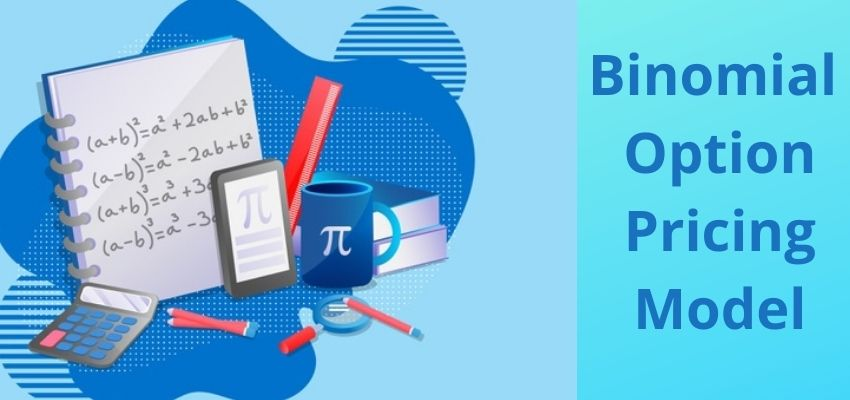# What is the Binomial Option Pricing Model?The binomial option pricing model is very simple to model that is used to price options. When to compared to the Black Scholes model and other complex models, the pricing model is mathematically simple and easy to use. This model is based on the concept of no-arbitrage. The binomial option is an important topic as far as FRM Part 1 exam is concerned. There are both conceptual and numerical questions in exams to test this topic. In this article, I will talk about various concepts related to the binomial option pricing model.

## Features of Binomial Option Pricing Model

• The pricing model values options using an iterative approach utilizing multiple periods to value American options.
• With the model, there are two possible outcomes with each iteration—a move up or a move down that follow a binomial tree.
• The model is intuitive and is used more frequently in practice than the well-known Black-Scholes model

## Assumptions in Binomial Option Pricing Model

The assumptions in this pricing models are as follows

1. There are only two possible prices for the underlying asset on the next day. From this assumption, this model has got its name as Binomial option pricing model (Bi means two)
2.  The two possible prices are the up-price and down-price
3. The underlying asset does not pay any dividends
4. The rate of interest (r) is constant throughout the life of the option
5. Markets are frictionless i.e. there are no taxes and no transaction cost
6. Investors are risk-neutral i.e. investors are indifferent towards risk

### Binomial options model building process

Let us consider that we have a share of a company whose current value is S0. Now in the next month, the price of this share is going to increase by u% (upstate) or it is going to go down by d% (downstate). No other outcome of price is possible for this stock in next month. Let p be the probability of upstate. Therefore the probability of downstate is 1-p.

Now let us assume that the call option exists for this stock which matures at the end of the month. Let the strike price of the call option be X. Now in case, the option holder decides to exercise the call option at the end of the month, what will be the payoffs?

The payoffs are given in the below diagram

Now, the expected payoff using the probabilities of upstate and downstate. From the above diagram, the expected value of the payoff is

Once the expected value of the payoff is calculated, this expected value of payoff has to be discounted by a risk-free rate to get the arbitrage-free price of the call option. Use continuous discounting for discounting the expected value of the payoff. FRM Part 1 uses continuous compounding and discounting for all numerical problems on derivatives.

In some questions, the probability of upstate is not given. In such a case, the probability of upstate can be calculated with the formula

Where;

p = up state probability

r = risk free rate

D = Down state factor

u = Up state factor

Using the above model building process, a similar model can be built for multi-period options and also for put options.

## Advantages of Binomial Option Pricing Model

1. The pricing models are mathematically simple to use.
2. The pricing model is useful for valuing American options in which the option owner has the right to exercise the option any time up till expiration.
3. The pricing model is also useful for pricing Bermudan options which can be exercised at various points during the life of the option.

## Limitations of Binomial Option Pricing Model

One major limitation of this pricing model is its slow speed. Computation complexity increases in the multi-period binomial option pricing model.